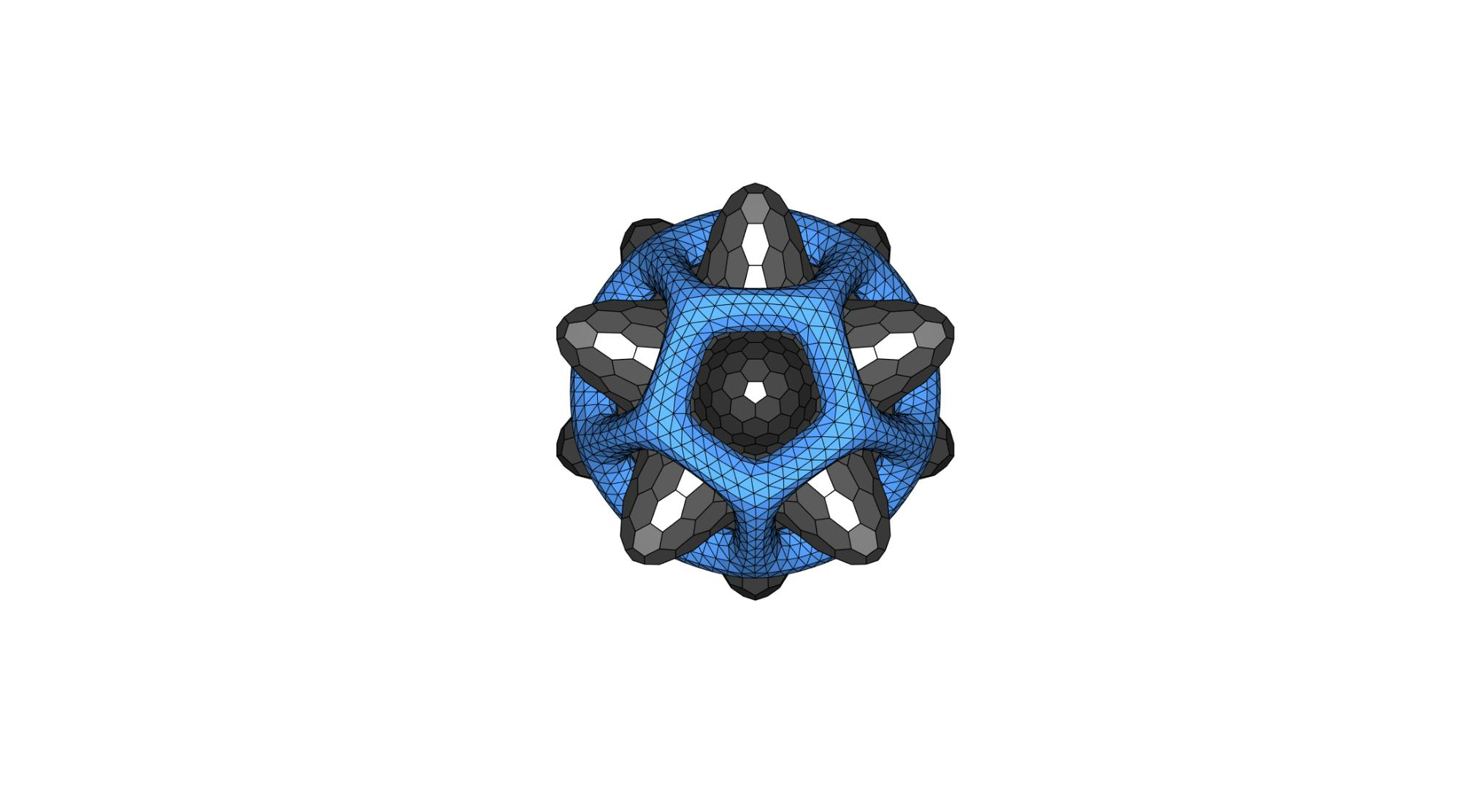# DEMO_JOSS_logo

Below is a demonstration for:

## Keywords

• platonic_solid, geoSphere, patch_dual, foamWrap
```clear; close all; clc;
```

## Plot settings

```patchColorInner=0.25*ones(1,3);
patchColorOuter=[64 120 192]/255;
```

## Control parameters

```rSphereInner=3; %Sphere radius
numRefine=3; %Number of refinement steps for geoSphere

smoothParInner.n=5;
smoothParInner.Method='LAP'; %Laplacian smoothing

smoothParOuter.n=50;
smoothParOuter.Method='HC'; %Humphreys-Classes smoothing

controlParFaomWrap.n=3;
controlParFaomWrap.dirFlip=1;
controlParFaomWrap.foamThickness=[]; %Empty uses default which is mean edgelength based
controlParFaomWrap.cParSmooth=smoothParOuter;
```

## Create inner shape

```% Create a sphere surface model
[Fs,Vs,~]=geoSphere(numRefine,rSphereInner); %Geodesic sphere triangulation

% Create an icosahedron surface model
[Vi,~]=platonic_solid(4,rSphereInner); %Get icosahedron

% Compute distances from the sphere to the icosahedron vertices
[D,indMin]=minDist(Vs,Vi);

D=D.^2; %Square distance
D=D-min(D(:)); D=D./max(D(:)); %Normalize [0 1]
D=D.*rSphereInner; %Multiply by radius [0 rSphere]

% Morph shape of sphere using distance data
[Ts,Ps,Rs] = cart2sph(Vs(:,1),Vs(:,2),Vs(:,3)); %Get spherical coordinates
[Vs(:,1),Vs(:,2),Vs(:,3)] = sph2cart(Ts,Ps,Rs_n); %Convert back to Cartesian coordinates
[Vs]=patchSmooth(Fs,Vs,[],smoothParInner); %Smoothen the result

%Compute the dual of the triangulation
[V_inner,F_inner]=patch_dual(Vs,Fs);
```

## Create rotation matrix

A rotation matrix is created to reorient the shapes such that one of the icosahedron vertices points at the camera

```n1=vecnormalize(Vi(1,:)); %Position vector 1 normalized to be axis 1
n2=vecnormalize(Vi(2,:)); %Position vector 2 normalized
n3=vecnormalize(cross(n1,n2)); %Axis 3 created as orthogonal to both n1 and n2
n2=vecnormalize(cross(n1,n3)); %Form axis 2 as orthogonal to both n1 and n3
Q=[n1; n2; n3]; %Create rotation matrix
```

## Create outer shape

First create an icosahedron, refine it, and compute the structure that wraps the surface.

```% Create refine icosahedron surface
[Vi,Fi]=platonic_solid(4,rSphereOuter); %Get icosahedron
[Fi,Vi,C,indIni]=triPolyDualRefine(Fi,Vi); %Refine the icosahedron

% Wrap surface
[F_outer,V_outer,C_outer,C_outer_c]=foamWrap(Fi,Vi,C,controlParFaomWrap);

% Keep only subset of surface components
F_outer=F_outer(C_outer_c~=1,:);
[F_outer,V_outer,~]=patchCleanUnused(F_outer,V_outer);
[F_outer,V_outer]=mergeVertices(F_outer,V_outer);
[F_outer,V_outer]=subtri(F_outer,V_outer,1); %Refine 1 iteration

%Smoothen to round the shape
[V_outer]=patchSmooth(F_outer,V_outer,[],smoothParOuter);
```

## Rotate geometries

```V_inner=V_inner*Q;
V_outer=V_outer*Q;
```

## Visualizaing surface models

A slightly messy plot (light is used twice to produce a bright look). Use the export figure widget to export images.

```hf=cFigure; hold on;

%Plot inner surface
for q=1:1:numel(F_inner)
hp=patch('Faces',F_inner{q},'Vertices',V_inner,'FaceColor',0.25*ones(1,3),'FaceAlpha',1,'lineWidth',1,'edgeColor','k');%0.25*ones(1,3));
end

%Plot outer surface
gpatch(F_outer,V_outer,patchColorOuter);

axisGeom; axis off;
view(0,0);
lighting flat;
drawnow;
```GIBBON www.gibboncode.org

Kevin Mattheus Moerman, [email protected]

GIBBON footer text

GIBBON: The Geometry and Image-based Bioengineering add-On. A toolbox for image segmentation, image-based modeling, meshing, and finite element analysis.

Copyright (C) 2006-2022 Kevin Mattheus Moerman and the GIBBON contributors

This program is free software: you can redistribute it and/or modify it under the terms of the GNU General Public License as published by the Free Software Foundation, either version 3 of the License, or (at your option) any later version.

This program is distributed in the hope that it will be useful, but WITHOUT ANY WARRANTY; without even the implied warranty of MERCHANTABILITY or FITNESS FOR A PARTICULAR PURPOSE. See the GNU General Public License for more details.

You should have received a copy of the GNU General Public License along with this program. If not, see http://www.gnu.org/licenses/.